# Matplotlib 101

Python 可視化中的經典

## plt.plot()

``import matplotlib.pyplot as plt``

`.plot()` 將繪製x與y軸上的線條或標記。`.show()` 是每一次都會用的方法，目的是將圖形輸出。

``````plt.plot([1, 2, 3])
plt.show()``````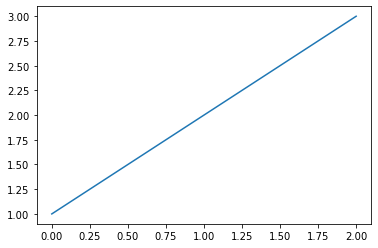``````plt.plot([1,2,3], [1,4,9])
plt.show()``````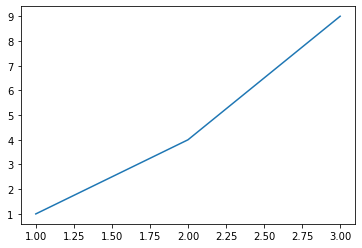## 自訂樣式

`matplotlib` 之所以強大是因為它有極為複雜可碩性極高的自訂功能。

### color 顏色

``````plt.plot([1,2,3,4], color='b')
plt.show()``````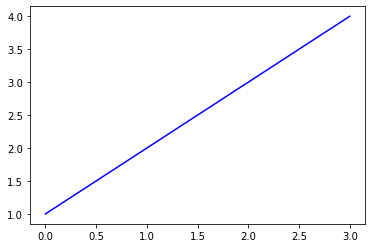### marker 標記

``````plt.plot([1,2,3,4], color='b', marker='^')
plt.show()``````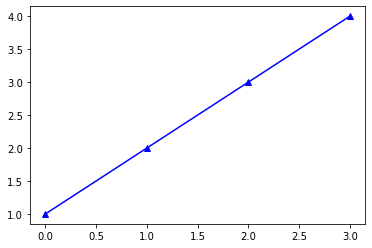Matplotlib 有極為方便的縮寫函數格式。以下我們將 顏色 和 標記樣式 更精簡的直接寫在一起。

``````plt.plot([1,2,3,4], 'b^')
plt.show()``````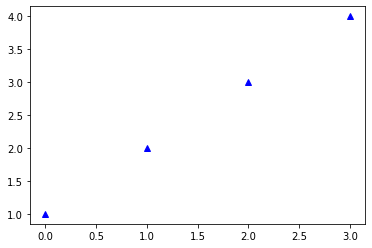``````plt.plot([1,2,3,4], 'ro')
plt.show()``````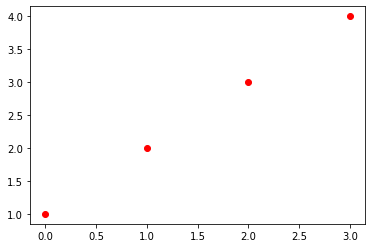### linestyle

``````plt.plot([1,2,3,4], 'gs', linestyle='dashed')
plt.show()``````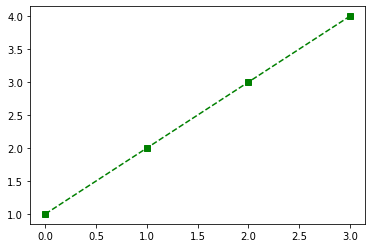``````plt.plot([1,2,3,4], 'gs', ls='dashed')
plt.show()````````````plt.plot([1,2,3,4], 'gs--')
plt.show()``````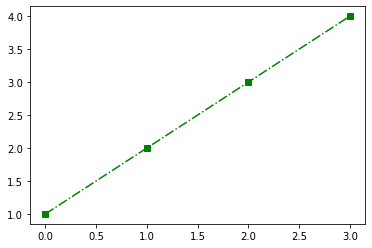``````plt.plot([1,2,3,4], 'gs-.')
plt.show()``````### linewidth & markersize

``````plt.plot([1,2,3,4], 'gs--', linewidth=2, markersize=12)
plt.show()``````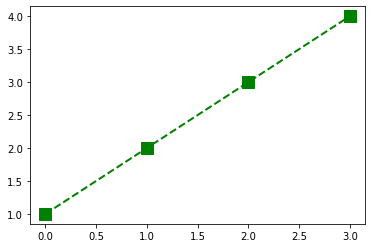`linewidth` 可縮寫成 `lw`

``````plt.plot([1,2,3,4], 'c:', lw=10, markersize=5)
plt.show()``````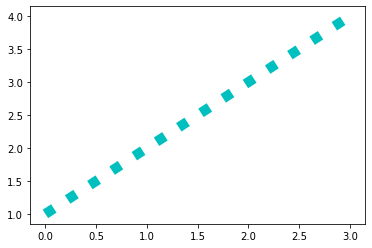### title, label & legend 標題、標籤和圖例

``````plt.plot([1,2,3,4], [1,2,3,4])
plt.title("No.1")
plt.show()``````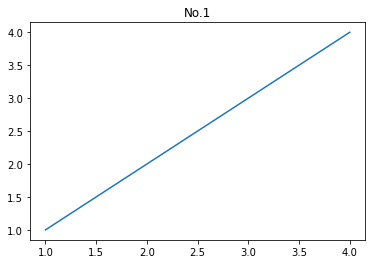``````plt.plot([1,2,3,4], [1,2,3,4])
plt.title("No.1")
plt.xlabel('Numbers')
plt.ylabel('Values')
plt.show()``````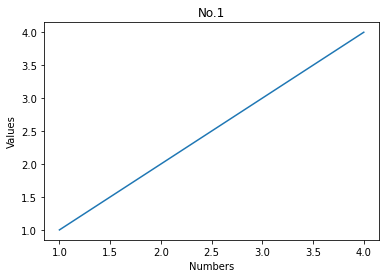``````plt.plot([1,2,3,4], [1,2,3,4])
plt.title("No.1")
plt.xlabel('Numbers')
plt.ylabel('Values')
plt.legend(['just data'])
plt.show()``````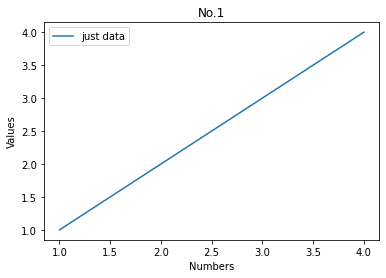## All together!

``````import numpy as np

x = np.arange(0., 5., 0.4)
x``````
`array([0. , 0.4, 0.8, 1.2, 1.6, 2. , 2.4, 2.8, 3.2, 3.6, 4. , 4.4, 4.8])`

``````plt.plot(x, x, x, x**2, x, x**3)
plt.show()``````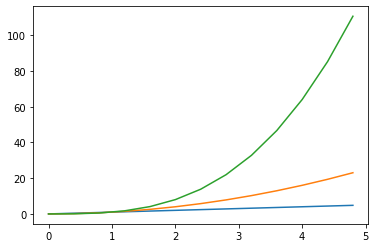``````plt.plot(x, x, 'b--', x, x**2, 'rs', x, x**3, 'g^')
plt.show()``````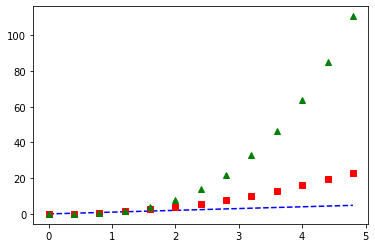``````plt.plot(x, x, 'b--', x, x**2, 'rs', x, x**3, 'g^')
plt.title("No.2")
plt.xlabel('Numbers')
plt.ylabel('Values')
plt.legend(['A','B','C'])
plt.show()``````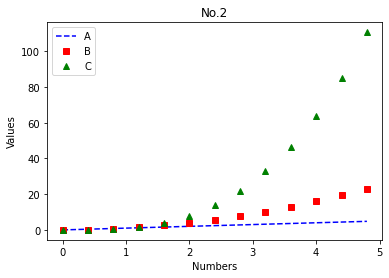v1.0